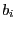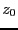Next: Coefficient of the characteristic Up: Weyl filter Previous: Mathematical background   Contents

Implementation

The Weyl filter is implemented to manage univariate polynomial (with numerical or interval coefficients) or parametric polynomial. A basic tool of this filter is

int Weyl_Filter_Utility(int Degree, double maxroot,
INTERVAL_VECTOR &b,INTERVAL_VECTOR &Input)
where Input(1) is the range for the polynom variable and b thecoefficients. A more general implementation is

int Weyl_Filter(int Degree, INTERVAL_VECTOR &Coeff,double maxroot,
int (* GetB)(int,INTERVAL_VECTOR &,INTERVAL &,INTERVAL_VECTOR &),
INTERVAL_VECTOR &Input)
Here GetB is a procedure that calculates thecoefficients. Its arguments are the degree of the polynomial, its coefficients,and thecoefficients. It should return 1 if the calculation has been successfull, 0 otherwise. An example of such procedure is Derive_Polynomial_Expansion. Degree is the degree of the polynomial, Coeff its coefficients and Input(1) is the range for the polynomial unknown.

This procedure has several variants:

int Weyl_Filter(int Degree, INTERVAL_VECTOR &Coeff,double max_root,
INTERVAL_VECTOR &Input)
here the GetB procedure will be Derive_Polynomial_Expansion. In

int Weyl_Filter(int Degree, INTERVAL_VECTOR &Coeff,
INTERVAL_VECTOR &Input)
here the GetB procedure will be Derive_Polynomial_Expansion and maxroot will be computed by an ALIAS procedure. The same procedure will be used in

int Weyl_Filter(int Degree, INTERVAL_VECTOR &Coeff,
int (* GetB)(int,INTERVAL_VECTOR &,INTERVAL &,INTERVAL_VECTOR &),
INTERVAL_VECTOR &Input)

Jean-Pierre Merlet 2012-12-20